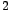DATA MINING
Desktop Survival Guide
by Graham Williams## Barplot

A barplot displays data as bars, each bar being proportional to the data being plotted. In R a barplot is built using the barplot function. We can use a barplot, for example, to illustrate the distribution of entities in a dataset across some variable. With the wine dataset Type is a categorical variable with three levels: 1, 2, and 3. A simple bar plot illustrates the distribution of the entities across the three Types. The summary function is used to obtain the data we wish to plot (59, 71, and 48).

We place the actual counts on the plot with the text function. The trick here is that the barplot function returns the bar midpoints, and these can be used to place the actual values. We addto the y values to place the numbers above the bars. Also note that xpd is set to TRUE to avoid the highest number being chopped (because it, 71, is actually outside the plot region).

[width=0.8]rplot-wine-barplot

 ```load("wine.Rdata") attach(wine) par(xpd=TRUE) bp <- barplot(summary(Type), xlab="Type", ylab="Frequency") text(bp, summary(Type)+2, summary(Type)) ```

http://rattle.togaware.com/code/rplot-wine-barplot.R